• 3d六边形网格笔筒模型：使用SolidWorks2014绘制；点击文章末尾的了解更多，观看视频讲解过程。绘制过程：1、在上视基准面上绘制草图 中心矩形；2、拉伸凸台：给定深度1；3、在前视基准面上绘制草图 六边形：中心点与...
3d六边形网格笔筒模型：使用SolidWorks2014绘制；点击文章末尾的了解更多，观看视频讲解过程。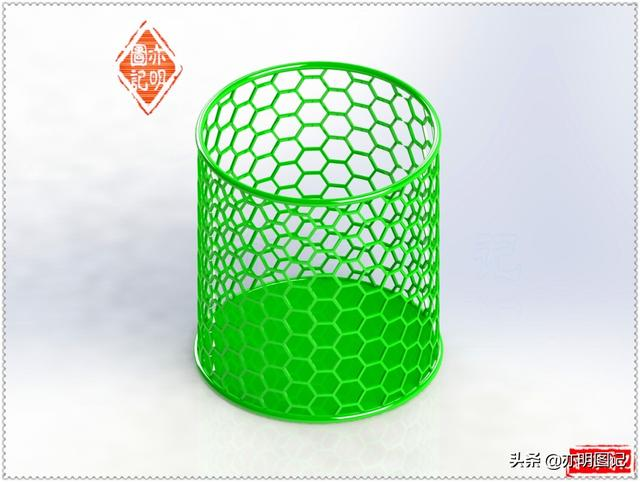绘制过程：1、在上视基准面上绘制草图 中心矩形；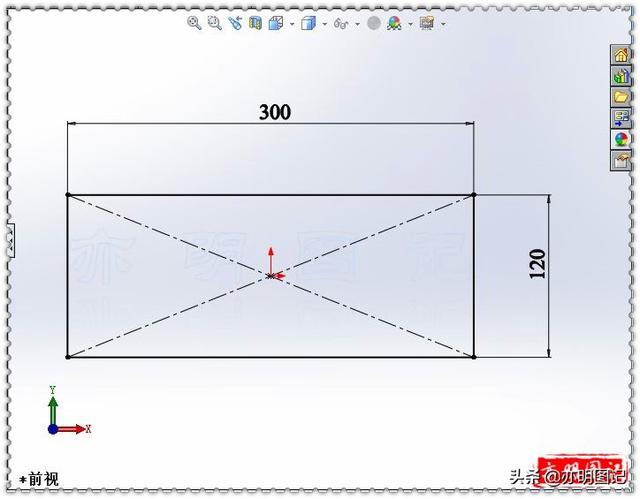2、拉伸凸台：给定深度1；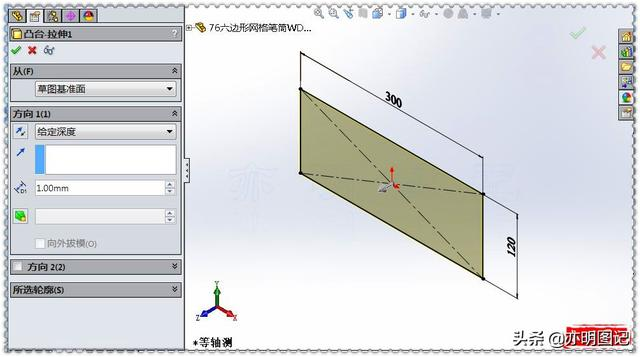3、在前视基准面上绘制草图 六边形：中心点与原点重合；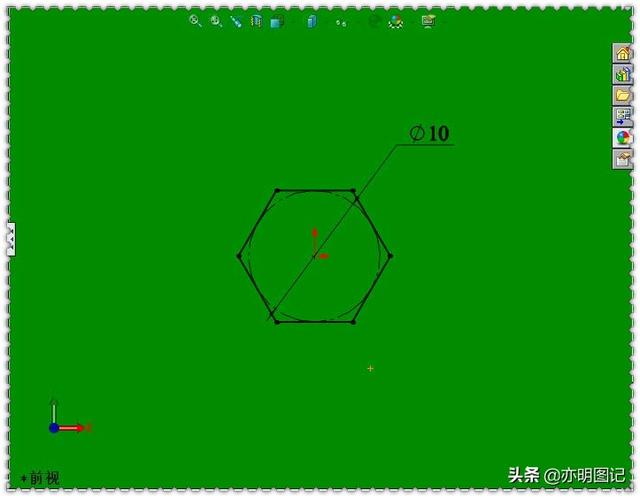4、拉伸切除：完全贯穿；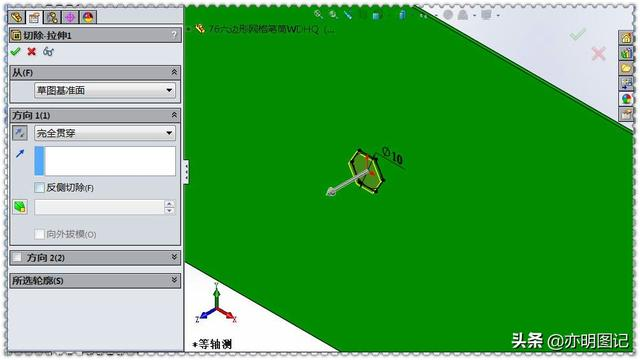5、填充阵列：填充边界选择前边的面；阵列布局选择穿孔；实例间距11；交错断续角度30°；阵列方向选择X轴方向的边线；要阵列的特征选择拉伸切除特征；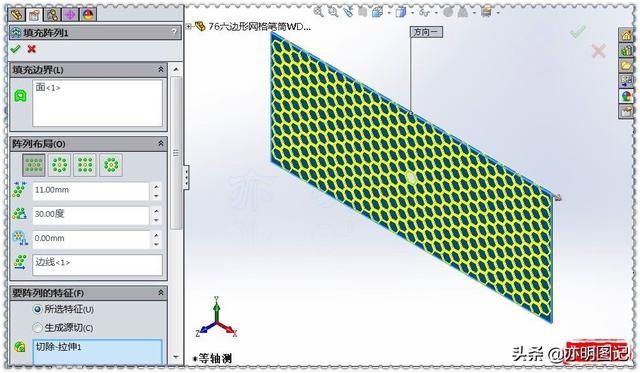6、在上视基准面上绘制草图 中心矩形；7、拉伸切除：完全贯穿；勾选反侧切除；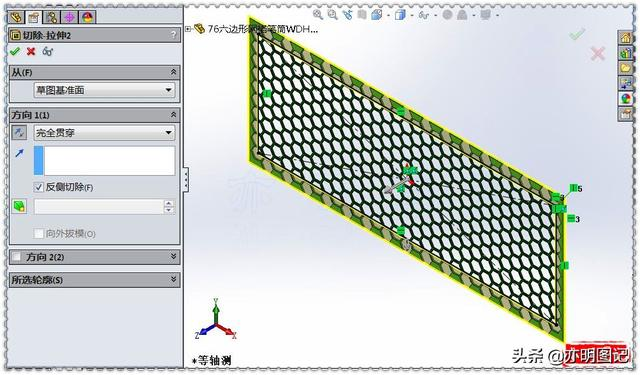8、弯曲：弯曲的实体选择网格实体；勾选折弯；角度360°；三重轴设定沿Y轴旋转角度90°；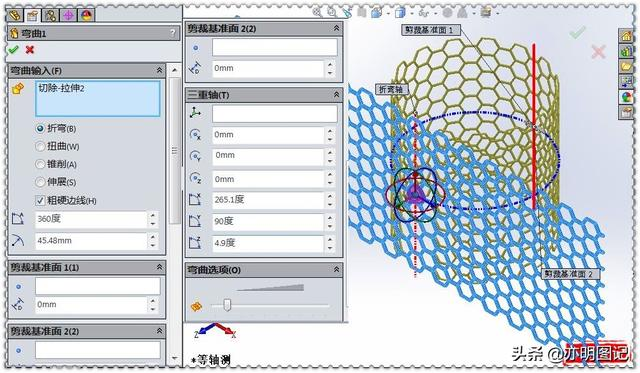9、在上视基准面上绘制草图 圆；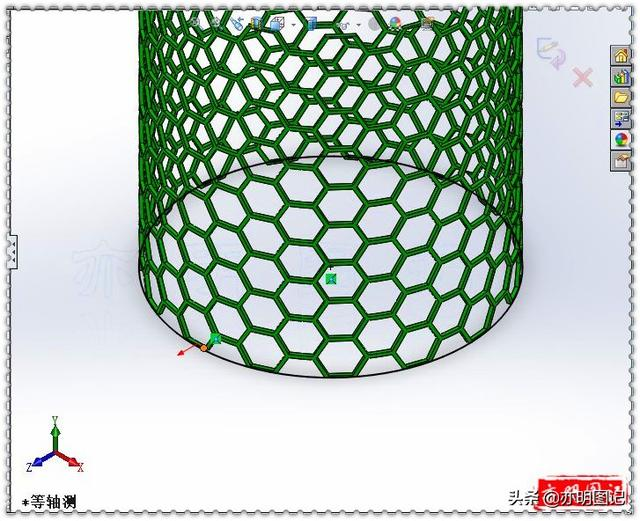10、拉伸凸台：两侧对称2；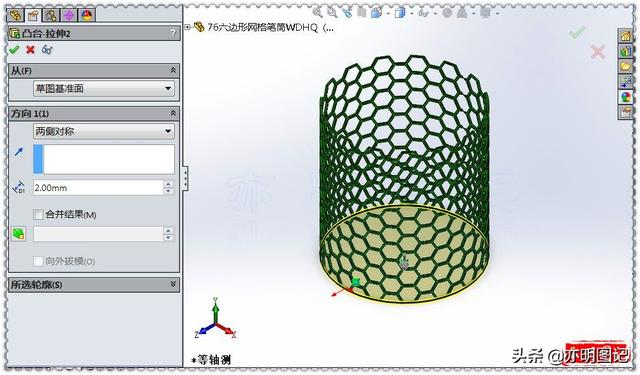11、在右视基准面上绘制草图 两个圆：直径3；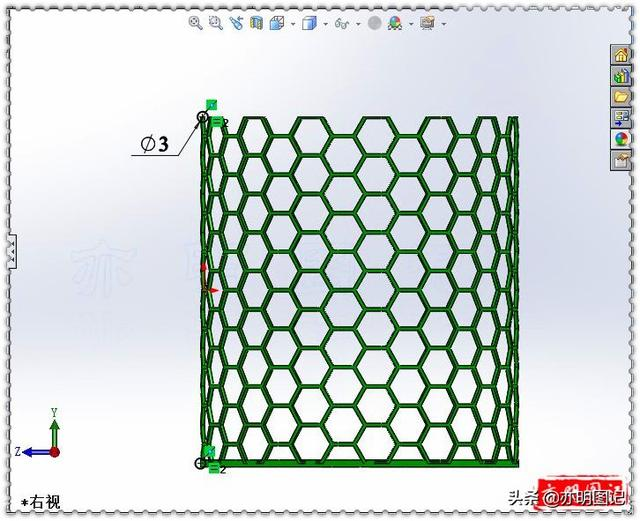12、旋转凸台：旋转轴选择底面拉伸凸台圆柱的基准轴；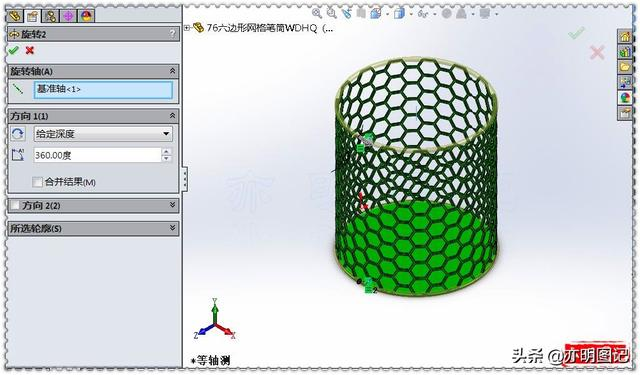13、完成。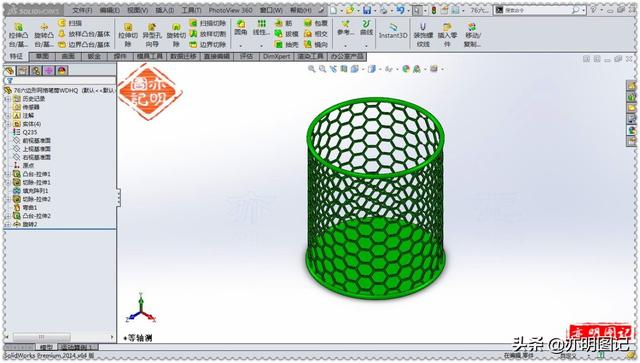点击关注@亦明图记 进入主页观看更多精彩内容。精彩回放：☞亦明图记：SolidWorks绘制花边果盘，如何绘制花边轮廓？投影曲线☞「亦明图记」SolidWorks绘制易拉罐，操作很简单，主要是草图绘制☞分享用SolidWorks绘制的网格圆环，路径怎么绘制，需借助另一实体☞亦明图记：SolidWorks绘制钟表，表盘数字的绘制用圆周阵列完成☞亦明图记：SolidWorks绘制果篮，用拉伸凸台切除绘制一个实体阵列点击"了解更多"，观看绘制该模型的视频讲解。↓↓↓
展开全文• 实现了六边形网格的向量工具，Hex为斜角坐标系，三个轴平面夹角为120度 NetHex为offset型坐标系，xy轴夹角90度 AxialHex为轴坐标系p,r夹角120度，支持互相转换Hex坐标系方便制作技能范围，寻路等功能 AxialHex...
实现了六边形网格的向量工具，

Hex为斜角坐标系，三个轴平面夹角为120度
NetHex为offset型坐标系，xy轴夹角90度
AxialHex为轴坐标系p,r夹角120度，支持互相转换

Hex坐标系方便制作技能范围，寻路等功能
AxialHex坐标系一般用于辅助计算
NetHex坐标系方便存储二维数组数据

同时支持坐标类型生成二维坐标（x,0,z）。后续会加入形状，距离等判断。用于制作复杂技能区域的游戏，并方便进行寻路计算。

图为Hex坐标系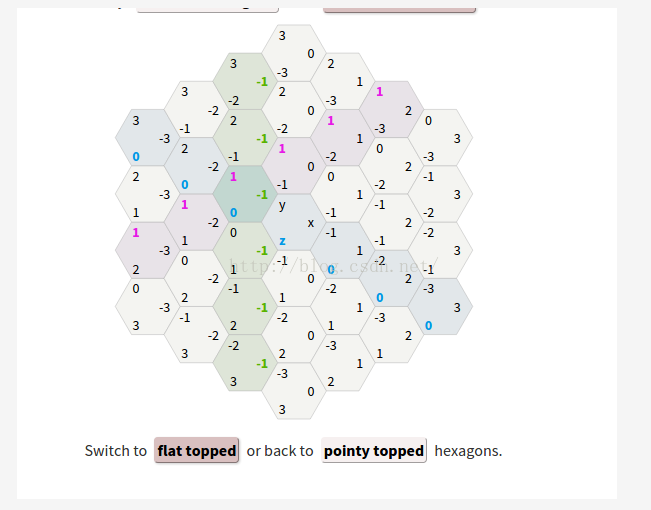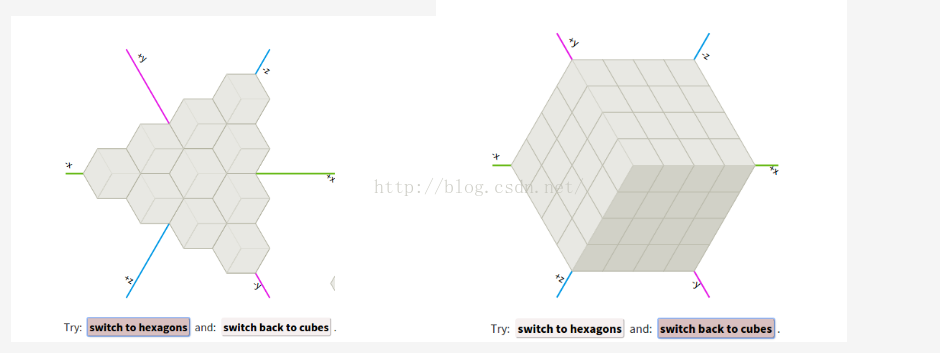图为几种不同的NetHex类型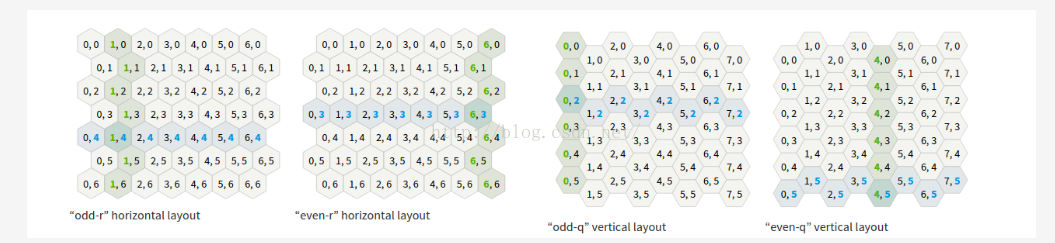具体数学原理参考redblobgames

下面是代码。

Hex.cs
提供基本数据格式

using System;
using System.Collections.Generic;
using UnityEngine;

namespace GridTool.Hex
{

public struct Hex
{
public int x;
public int y;
public int z;

public Hex(int x, int y, int z)
{
this.x = x;
this.y = y;
this.z = z;
}

public int this[int index]
{
get
{
switch (index)
{
case 0:
return x;
case 1:
return y;
case 2:
return z;
default:
throw new IndexOutOfRangeException("Invalid Hex index!");
}
}
set
{
switch (index)
{
case 0:
x = value;
break;
case 1:
y = value;
break;
case 2:
z = value;
break;
default:
throw new IndexOutOfRangeException("Invalid HexTransform index!");
}
}
}

//========== 快速构造 ==========

/// <summary>
/// 原点
/// </summary>
public static Hex zero
{
get
{
return new Hex(0, 0, 0);
}
}

/// <summary>
/// 左上
/// </summary>
public static Hex LTop
{
get
{
return new Hex(0, 1, -1);
}
}

/// <summary>
/// 右上
/// </summary>
public static Hex RTop
{
get
{
return new Hex(1, 0, -1);
}
}
public static Hex Left
{
get
{
return new Hex(-1, 1, 0);
}
}
public static Hex Right
{
get
{
return new Hex(1, -1, 0);
}
}
public static Hex LBottom
{
get
{
return new Hex(-1, 0, 1);
}
}
public static Hex RBottom
{
get
{
return new Hex(0, -1, 1);
}
}

public static Hex[] Directions
{
get
{
return new Hex {
Hex.LTop,
Hex.RTop,
Hex.Right,
Hex.RBottom,
Hex.LBottom,
Hex.Left
};
}
}

//========== 数组操作 =========
public static Hex[] operator +(Hex[] a, Hex b)
{
Hex[] c = new Hex[a.Length];
for (int i = 0; i < a.Length; i++)
{
c[i] = a[i] + b;
}
return c;
}

public static Hex[] operator -(Hex[] a, Hex b)
{
Hex[] c = new Hex[a.Length];
for (int i = 0; i < a.Length; i++)
{
c[i] = a[i] - b;
}
return c;
}

//========== 运算符 =========

public static Hex operator +(Hex a, Hex b)
{
return new Hex(a.x + b.x, a.y + b.y, a.z + b.z);
}

public static Hex operator -(Hex a, Hex b)
{
return new Hex(a.x - b.x, a.y - b.y, a.z - b.z);
}

public static Hex operator -(Hex a)
{
return new Hex(-a.x, -a.y, -a.z);
}
public static Hex operator *(Hex a, int d)
{
return new Hex(a.x * d, a.y * d, a.z * d);
}

public static Hex operator *(int d, Hex a)
{
return new Hex(a.x * d, a.y * d, a.z * d);
}

public static Hex operator /(Hex a, int d)
{
return new Hex(a.x / d, a.y / d, a.z / d);
}

public static bool operator ==(Hex lhs, Hex rhs)
{
return lhs.x == rhs.x && lhs.y == rhs.y && lhs.z == rhs.z;
}

public static bool operator !=(Hex lhs, Hex rhs)
{
return lhs.x != rhs.x || lhs.y != rhs.y || lhs.z != rhs.z;
}

//========== 工具 =========
public static int Distance(Hex from, Hex to)
{
return (int)Mathf.Max(Mathf.Abs(from.x - to.x), Mathf.Abs(from.y - to.y), Mathf.Abs(from.z - to.z));
}

public override string ToString()
{
return String.Format("({0}, {1}, {2})", x, y, z);
}
public string ToString(string format)
{
return String.Format("({0}, {1}, {2})", x.ToString(format), y.ToString(format), z.ToString(format));
}

public void Fix()
{
y = -x - z;
}

}

public struct AxialHex
{
public int q;
public int r;
public AxialHex(int q, int r)
{
this.q = q;
this.r = r;
}

public static AxialHex RoundAxial(float q, float r)
{
return HexTools.RoundHex(q, -q - r, r).ToAxial();
}
}

public enum HexType
{
Odd_r,// 行对齐 偶数行偏左
Even_r,// 行对齐 偶数行偏右
Odd_q,// 列对齐 0
Even_q,//
NULL//
}

public struct NetHex
{
public int col;
public int row;

public NetHex(int col, int row)
{
this.col = col;
this.row = row;

}

public override string ToString()
{
return String.Format("({0}, {1})", col, row);
}
}
}

工具类
处理各种坐标系之间的转换关系等。

using System;
using System.Collections;
using System.Collections.Generic;
using UnityEngine;

namespace GridTool.Hex
{
public static class HexTools
{
public static Hex ToHex(this AxialHex ax)
{
return new Hex(ax.q, -ax.q - ax.r, ax.r);
}

public static AxialHex ToAxial(this Hex hex)
{
return new AxialHex(hex.x, hex.z);
}

public static Hex ToHex(this NetHex grid, HexType type)
{
Hex hex = new Hex(0, 0, 0);
int col = grid.col;
int row = grid.row;
switch (type)
{
case HexType.Odd_q:
{
hex.x = col;
hex.z = row - (col - (col & 1)) / 2;
hex.y = -hex.x - hex.z;
}
break;
case HexType.Even_q:
{
hex.x = col;
hex.z = row - (col + (col & 1)) / 2;
hex.y = -hex.x - hex.z;
}
break;
case HexType.Odd_r:
{
hex.x = col - (row - (row & 1)) / 2;
hex.z = row;
hex.y = -hex.x - hex.z;
}
break;
case HexType.Even_r:
{
hex.x = col - (row + (row & 1)) / 2;
hex.z = row;
hex.y = -hex.x - hex.z;
}
break;
default:
break;
}
return hex;
}

public static NetHex ToNet(this Hex hex, HexType type)
{
int col;
int row;
switch (type)
{
case HexType.Odd_q:
{
col = hex.x;
row = hex.z + (hex.x - (hex.x & 1)) / 2;
}
break;
case HexType.Even_q:
{
col = hex.x;
row = hex.z + (hex.x + (hex.x & 1)) / 2;
}
break;
case HexType.Odd_r:
{
col = hex.x + (hex.z - (hex.z & 1)) / 2;
row = hex.z;
}
break;
case HexType.Even_r:
{
col = hex.x + (hex.z + (hex.z & 1)) / 2;
row = hex.z;
}
break;
default:
{
col = 0;
row = 0;
}
break;
}
return new NetHex(col, row);
}

//类型是否尖角朝上
public static bool IsPointTop(this HexType type)
{
return type == HexType.Even_r || type == HexType.Odd_r;
}

//网格中心相对0点便宜位置，y轴为0。
public static Vector3 ToPixel(this Hex hex, float size, HexType type)
{

AxialHex ax = hex.ToAxial();
if (IsPointTop(type))
return new Vector3(
size * 1.73205080756887f * (ax.q + ax.r * .5f),
0,
size * 1.5f * ax.r);
else
return new Vector3(size * 1.5f * ax.q,
0,
size * 1.73205080756887f * (ax.r + ax.q * .5f));
}

public static Hex ToHex(this Vector3 pixel, float size, HexType type)
{
float q = 0;
float r = 0;
if (IsPointTop(type))
{
q = ((pixel.x * 1.73205080756887f - pixel.z) / (size * 3));
r = (pixel.z * 0.666666666666f / size);
}
else
{
q = (pixel.x * 0.666666666666f / size);
r = ((pixel.z * 1.73205080756887f - pixel.x) / (size * 3));
}
AxialHex ax = AxialHex.RoundAxial(q, r);
return ax.ToHex();
}

public static Hex RoundHex(float x, float y, float z)
{
float rx = Mathf.Round(x);
float ry = Mathf.Round(y);
float rz = Mathf.Round(z);

float x_diff = Mathf.Abs(rx - x);
float y_diff = Mathf.Abs(ry - y);
float z_diff = Mathf.Abs(rz - z);

if (x_diff > y_diff && x_diff > z_diff)
rx = -ry - rz;
else if (y_diff > z_diff)
ry = -rx - rz;
else
rz = -rx - ry;

return new Hex((int)rx, (int)ry, (int)rz);
}

/// <summary>
/// 获取相邻坐标组，左上顺时针旋转
/// </summary>
/// <param name="hex">目标坐标</param>
/// <returns>坐标组</returns>
public static Hex[] GetNeighbor(this Hex hex)
{
return Hex.Directions + hex;
}

/// <summary>
/// 获取经过两点之间的坐标组
/// </summary>
/// <param name="from">开始坐标</param>
/// <param name="target">结束坐标</param>
/// <returns>坐标组</returns>
public static Hex[] LineTo(this Hex from, Hex target)
{
int N = Hex.Distance(from, target);
Hex[] results = new Hex[N];

for (int i = 0; i <= N; i++)
{
float t = (float) i / (float) N;
results[i] = HexTools.RoundHex(Mathf.Lerp(from.x,target.x,t), Mathf.Lerp(from.y,target.y,t), Mathf.Lerp(from.z,target.z,t));
}
return results;
}

/// <summary>
/// 获取中心点范围内的所有坐标
/// </summary>
/// <param name="center">中心点</param>
/// <returns>坐标组</returns>
public static Hex[] Range(this Hex center, int radius)
{
List<Hex> results = new List<Hex>();
{
{
int dz = -dx - dy;
}
}
return results.ToArray();
}

/// <summary>
/// </summary>
/// <param name="center">中心位置</param>
/// <returns>环形包含的格子</returns>
public static Hex[] Ring(this Hex center, int radius)
{
List<Hex> results = new List<Hex>();

Hex tmp = center + Hex.Directions * radius;
for (int i = 0; i < 6; i++)
{
for (int j = 0; j < radius; j++)
{
tmp = tmp.GetNeighbor()[i];
}
}
return results.ToArray();
}

}
}
展开全文• 也就是正六边形，也就是以下这个东东：一般的问答模式例如以下：亲们问：ArcGIS里面那个工具能够做这个东东？虾神答：额，没有原生的工具。亲们问：那我看非常多人都做了啊，这个东东怎么做？虾神答：嗯，算法非常...
近来有不少同学。都有问我关于蜂窝多边形的问题。也就是正六边形，也就是以下这个东东：一般的问答模式例如以下：亲们问：ArcGIS里面那个工具能够做这个东东？虾神答：额，没有原生的工具。亲们问：那我看非常多人都做了啊，这个东东怎么做？虾神答：嗯，算法非常easy……巴拉巴拉巴拉……亲们：虾神：……好吧，既然有如此，今天我们就用一篇文章来简单说说在ArcGIS里面怎样做蜂窝六边形的方法。话说我们做样方分析的时候，最简单的当然是採用矩形。样方样方……里面这个方字用得那是极好的。可是非常多精益求精的同学，认为方形太图样图森破了，我们怎么能用这么简单的东西呢？我们要用正六边形！实际上，六边形得几何描写叙述确实要例如形完美得多，对照一下就知道了：首先在几何里面有“平面镶嵌”这个概念(无间隙且不重叠地覆盖平面)，正六边形是边数最多的平面镶嵌图形。(边越多，越接近理论上的完美图形——圆)然后正六边形延伸距离都是一样的。当然，还有很多其它的优点，我就不列举了。以下进入正题。我们来说说，怎样在ArcGIS里面做出正六边形来。做法例如以下：1、首先用ArcGIS里面的渔网工具生成渔网网格：注意，渔网生成工具，在10.4之前，位置在：ArcToolbox ——Data Management Tools ——Feature Class工具箱以下可是在10.4之后，位置移动到了：ArcToolbox ——Data Management Tools ——Sampling 工具箱以下在生成渔网的时候。一定要注意一个细节问题：也就是高度宽度比，一定是1.5:1。假设不这样设置，那么你的六边形就会变成四边形了……切记切记。另外我们使用的是Label Point(也就是渔网的中心点)。生成完毕之后。将这个渔网的中心点复制一份，例如以下：然后把label2移动到label1的对角中心位置上去，能够用渔网的交点进行參考校正。例如以下：移动完毕之后，变成了以下这个样子：接下去，把这个两个点图层合并起来，合并的工具用Merge或者append都能够，自选，合并完毕之后。把这个合并完毕的点图层。作为泰森多边形的中心点，生成太泰森多边形。例如以下：泰森多边形工具在ArcToolbox —— Analysis Tools —— Proximity —— Create Thiessen Polygons生成完毕之后。例如以下：然后把周边多余的部分裁掉：蜂窝多边形到此完毕……这一系列过程，能够通过脚本来实现，工具都已经给出了，有兴趣的同学能够自己写脚本来做。有下面几个地方须要注意：1、生成渔网得时候。须要大于你要覆盖的区域。依据80/20原则，你生成的渔网得extent最好大于你区域的20%左右。2、渔网的高度和宽度比，大约在1:1.5左右，这个比例依据空间參考的不同。能够适当的微调。3、最好用投影坐标系。4、假设格网窗体太小。那么生成的渔网太多，会非常慢非常慢。当然，里面还挖了一个坑……就是泰森多边形的算法，这个东东是我见过的最简单粗暴的算法……灰常灰常简单，有空我们再说了。蜂窝多边形的脚本工具，有须要的能够给我发邮件：[email protected]。
展开全文• 我想使用XYZ坐标创建一个六边形网格，它是以螺旋形模式构建的。这是我当前的代码，它生成一个由下面的红色箭头描述的网格。我的问题区域被圈起来了。我需要从[-1,0,1]移动到[-1,0,1]到[-1，-1,2](沿着蓝线)。在完整...
我想使用XYZ坐标创建一个六边形网格，它是以螺旋形模式构建的。这是我当前的代码，它生成一个由下面的红色箭头描述的网格。我的问题区域被圈起来了。我需要从[-1,0,1]移动到[-1,0,1]到[-1，-1,2](沿着蓝线)。在完整的代码显示在哈希行下面-我正在Blender2.65a中创建可视化radius = 11 # determines size of fielddeltas = [[1,0,-1],[0,1,-1],[-1,1,0],[-1,0,1],[0,-1,1],[1,-1,0]]hex_coords = []for r in range(radius):x = 0y = -rz = +rpoints = x,y,zhex_coords.append(points)for j in range(6):if j==5:num_of_hexas_in_edge = r-1else:num_of_hexas_in_edge = rfor i in range(num_of_hexas_in_edge):x = x+deltas[j]y = y+deltas[j]z = z+deltas[j]plot = x,y,zhex_coords.append(plot)-#-#-#-#-#-#-#-#-#-#-#-#-#-#-#-#-#-#-#-#-#-#-#-#-#-在^{pr2}\$
展开全文• 本篇博文为博主(whgiser)原创，...六边形可以说其几何描述非常完美，但Arcgis并没有提供直接的工具生成六边形。受博文启发，该博文阐述了利用泰森多边形方法生成蜂窝，但是文章中没讲具体如何去算长度，只是基于大...
• 我找到了帮助我生成坐标的this问题，并稍微修改了代码，将所有坐标作为键存储在一个值为“.”(floor)或“X”(wall)的字典中，还包含了一个函数，该函数打印出一个表示地图的字符串，其中每个非空字符表示一个六边形...
• 生成19个六边形分布的网格模型，每个六边形的中心点即为BS的位置，默认1号为原点。在一号BS范围内，生成随机的UE，通过筛选，让所有生成的点落在1号的六边形区域内。
• 1.基础六边形网格   基础网格很容易绘制，六个边缘点，一个中心点，如何在引擎中绘制动态网格网上一搜一大把，这里就不介绍了。 2.边缘扰动   方方正正的网格看起来太拘束，利用一张噪声图或者其他方式给六边形...unity
• 它承载了一个非凡的程序，能够始终如一地生成高质量的完整六边形网格，适用于有限元分析。 Hexi的文档分为三部分，可以在此处进行检查：https://hexi.sourceforge.io/Hexidoc_Part1_210420.pdf、...开源软件
• 基于ghostcat工具类的AStar算法改编而成,非6方向的网格寻路也可以使用； 专用于六边形/六方向寻路,使用时请注意不要与原本的4/8方向方法混淆； 地图为二维数组，示例为生成的地图为左右交错、简单直观的展示了基本...
• H3是一种使用六边形网格的地理空间索引系统，该六边形网格可以（近似）细分为越来越细的六边形网格，将六边形网格的优点与的层次细分相结合。 可从获得文档。 可在目录下找到Markdown格式的开发人员文档。 将错误...
• Unity插件，用于在Terrain或模型上生成六边形网格，用来做RTS策略游戏很方便
• Unity插件，用于在Terrain或模型上生成六边形网格，用来做RTS策略游戏很方便 Terrain Grid System V9.0.2 用于生成各种网格，支持四边形、六边形、不规则形状的网格，自带寻路算法，网格可以依附3D地形表面，RTS...
• 在应该有圆角的地方我们都简化了处理，目的就是为了生成好的网格， 另外建议用sw画不要用ug画几何体，因为ug的圆柱侧面会产生两个版面，这不利于我们后续的约束的添加。 本人在sw中画的装配体： 然后把装配体...ansys
• 该软件用arcmap的工具箱加载。可以生成用户指定的正六边形网格叠加在底图(矢量或者栅格)上，用于后续的分析。
• 您从8 x 5的六边形网格开始 每个六边形在每个边缘上具有4种颜色之一 您可以左键单击任意给定的六边形逆时针旋转60度，右键单击以顺时针旋转60度 您从时钟开始计时100秒 如果菱形与4个六边形接壤的菱形的所有4条边都...
• 在了解的过程中又发现，战旗，三国志这种类型的游戏好像都是采用的自动网格，其中包含六边形网格。这让我慢慢的感兴趣起来了。在逐渐摸索过程中，发现，这个还是很有意思的，哈哈。学习之路，大mesh unity
• 如果数字看起来不太有趣，请尝试在正方形和六边形网格之间切换。 您可以预测下次单击“执行”按钮时会发生什么情况吗？ 您能弄清楚为什么会出现分形图案吗？ CloisterWalk可以用来测试数字的非理性吗？ 享受！开源软件
• 自动导航网格生成可避免您手动执行。完全多线程，因此几乎不会影响帧速率。使用光线投射，平滑和使用漏斗算法进行路径后处理。寻路调用的一行代码。图形可以保存到文件中。在XZ和XY平面上均进行局部回避。包含源代码...
• Terrain Grid System V9.0.2 用于生成各种网格，支持四边形、六边形、不规则形状的网格，自带寻路算法，网格可以依附3D地形表面，RTS开发的利器。 仅供个人研究使用，请勿商用，否则后果自负。
• 有限元模型建立方法是：先创建正六边形，和扫略线条，对正六边形用一根直线划分为两个四边形，从而可以进行四边形映射网格划分。划分好之后，利用extrude将划分好的六边形有限面单元扫描成扳手，从而生成有限元模型...
• 1.简介 ...开发者们发明了多种不同的方法来解决抽象图数据的问题，例如2D空间下的基于不同形状（正方形、六边形）的网格，3D空间下的基于不同多边形的网格，可视图，路点图等等。 而另一方便，在解决路算法
• -六边形网格和寻径支持：完全交互式网格（选择，着色，纹理） 还有更多。。。 -三类城市分类，包括国家和地区首府。 -数据包括：大陆、国家、城市和省份名称、关系、每个国家和省的多个地区、大都市人口、...
• 同样可以生成相应的源代码，不再赘述；主要进行plots分析； SOM simple hits SOM的默认拓扑是六边形。这个数字显示了拓扑中的神经元位置,并指出了多少训练数据与每个神经元(集群中心)相关联。拓扑是一个10×10的网格...神经网络 matlab
• 8.6.2 hextop——六边形拓扑函数/252 8.6.3 randtop——随机拓扑结构函数/253 8.6.4 tritop——三角拓扑函数/253 8.6.5 dist、boxdist、linkdist、mandist——距离函数/255 8.6.6 newc——竞争网络/258 8.6.7 ...
• #设置六边形区域分割网格，分别设置X，Y值的偏移量 k = math.sqrt(3) / 2 # Vertical offset for hexagonal grid cells x_min = berlin_center_x - 6000 x_step = 600 y_min = berlin_center_y - 6000 - (int(21...python
• , 新增统计变换函数stat_summary2d()（在二维网格上计算数据密度），stat_summary_hex()（在六边形“蜂巢”上计算数据密度），stat_bindot()（一维点图密度），stat_ydensity()（密度曲线，用于小提琴图）。...
• 4.创建正六边形 2.5.5 草绘实例5 2.6本章小结 2.7过关练习题 第3章 建模基础 3.1 基本概念 3.2常用的基准特征 3.2.1 基准平面 1.基准平面概述 2.创建基准平面实例 3.基准特征显示与隐藏 3.2.2基准轴 3.2.3基准点 1....